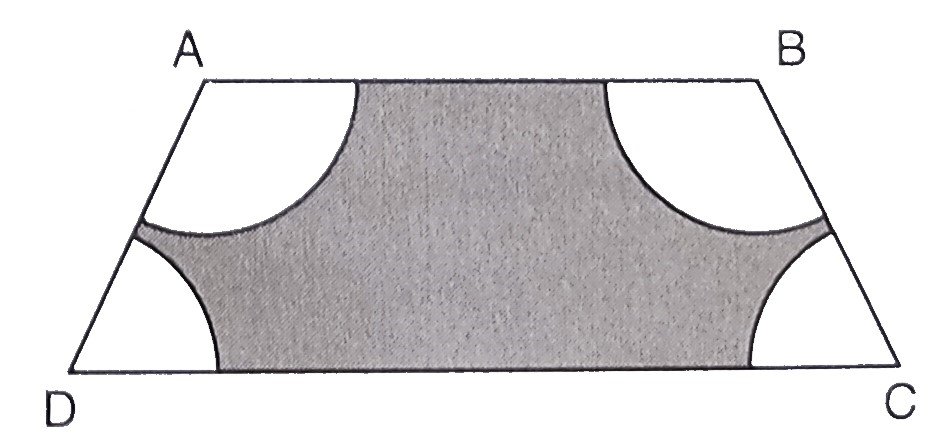"
">

# In the figure below, $A B C D$ is a trapezium with $A B \| D C, A B=18 \mathrm{~cm}, D C=32 \mathrm{~cm}$ and the distance between $A B$ and $D C$ is $14 \mathrm{~cm}$. Circles of equal radii $7 \mathrm{~cm}$ with centres $A, B, C$ and $D$ have been drawn. Then, find the area of the shaded region of the figure. (Use $\pi=22 / 7)$."

Given:

$A B C D$ is a trapezium with $A B \| D C, A B=18 \mathrm{~cm}, D C=32 \mathrm{~cm}$ and the distance between $A B$ and $D C$ is $14 \mathrm{~cm}$.

Circles of equal radii $7 \mathrm{~cm}$ with centres $A, B, C$ and $D$ have been drawn.

To do:

We have to find the area of the shaded region of the figure.

Solution:

Radius of each sector at the corner of the trapezium $= 7\ cm$

Sum of the angles of a quadrilateral $=360^{\circ}$

This implies,

The four sectors complete a circle.

Therefore,

Area of the circle $=\pi(7)^{2}$

$=\frac{22}{7} \times 49$

$=154 \mathrm{~cm}^{2}$

Area of the trapezium $=\frac{1}{2}(\mathrm{AB}+\mathrm{DC}) \times h$

$=\frac{1}{2}(18+32) \times 14$

$=\frac{1}{2} \times 50 \times 14$

$=350 \mathrm{~cm}^{2}$

Therefore,

Area of the shaded region $=$ Area of the trapezium $-$ Sum of the areas of the four sectors

$=350-154$

$=196 \mathrm{~cm}^{2}$

The area of the shaded region is $196\ cm^2$.

Updated on: 10-Oct-2022

16 Views